GFG App
Open AppBrowser
Continue

# Evaluation of Postfix Expression

Given a postfix expression, the task is to evaluate the postfix expression.

Postfix expression: The expression of the form “a b operator” (ab+) i.e., when a pair of operands is followed by an operator.

Examples:

Input: str = “2 3 1 * + 9 -“
Output: -4
Explanation: If the expression is converted into an infix expression, it will be 2 + (3 * 1) – 9 = 5 – 9 = -4.

Input: str = “100 200 + 2 / 5 * 7 +”
Output: 757

## Evaluation of Postfix Expression using Stack:

To evaluate a postfix expression we can use a stack.

Iterate the expression from left to right and keep on storing the operands into a stack. Once an operator is received, pop the two topmost elements and evaluate them and push the result in the stack again.

Illustration:

Follow the below illustration for a better understanding:

Consider the expression: exp = “2 3 1 * + 9 -“

• Scan 2, it’s a number, So push it into stack. Stack contains ‘2’.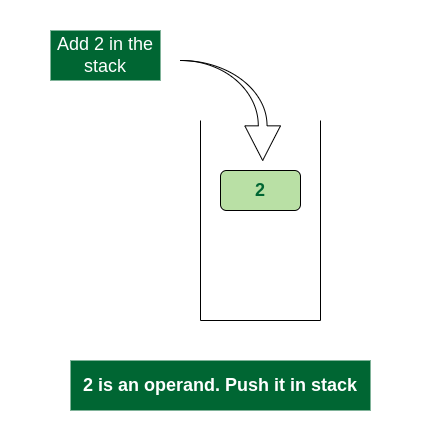Push 2 into stack

• Scan 3, again a number, push it to stack, stack now contains ‘2 3’ (from bottom to top)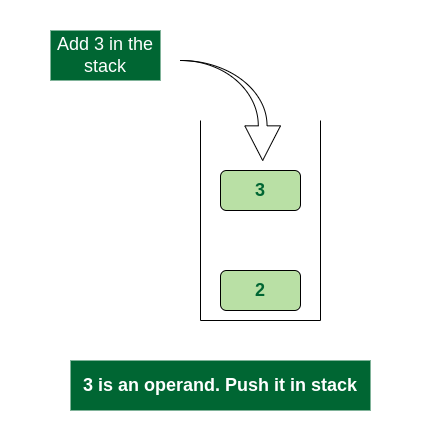Push 3 into stack

• Scan 1, again a number, push it to stack, stack now contains ‘2 3 1’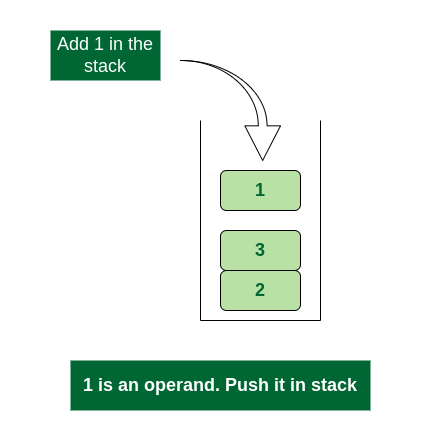Push 1 into stack

• Scan *, it’s an operator. Pop two operands from stack, apply the * operator on operands. We get 3*1 which results in 3. We push the result 3 to stack. The stack now becomes ‘2 3’.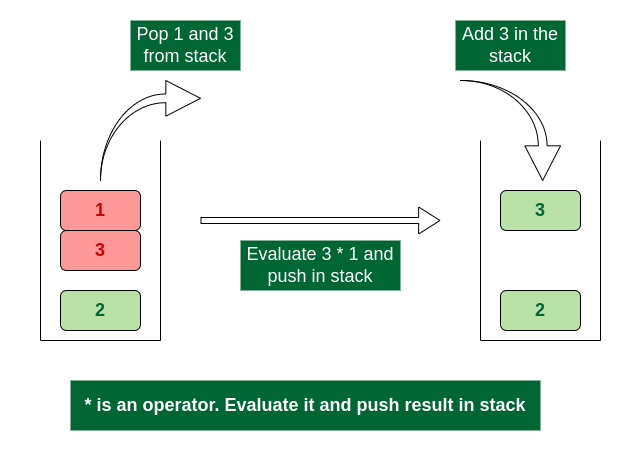Evaluate * operator and push result in stack

• Scan +, it’s an operator. Pop two operands from stack, apply the + operator on operands. We get 3 + 2 which results in 5. We push the result 5 to stack. The stack now becomes ‘5’.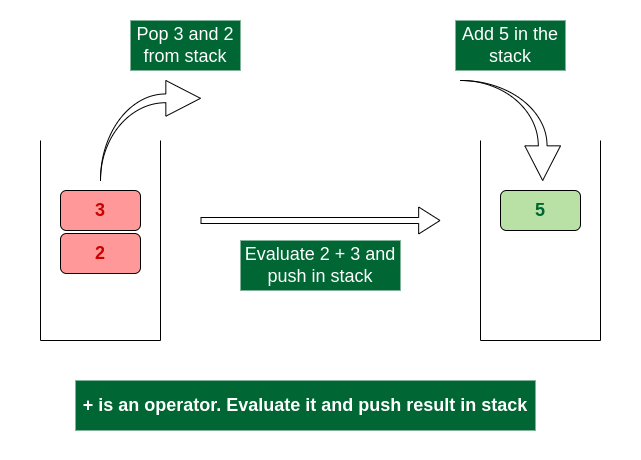Evaluate + operator and push result in stack

• Scan 9, it’s a number. So we push it to the stack. The stack now becomes ‘5 9’.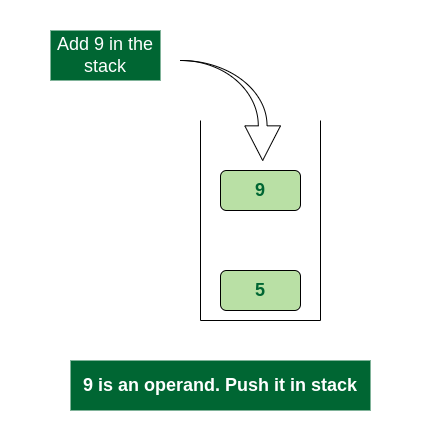Push 9 into stack

• Scan -, it’s an operator, pop two operands from stack, apply the – operator on operands, we get 5 – 9 which results in -4. We push the result -4 to the stack. The stack now becomes ‘-4’.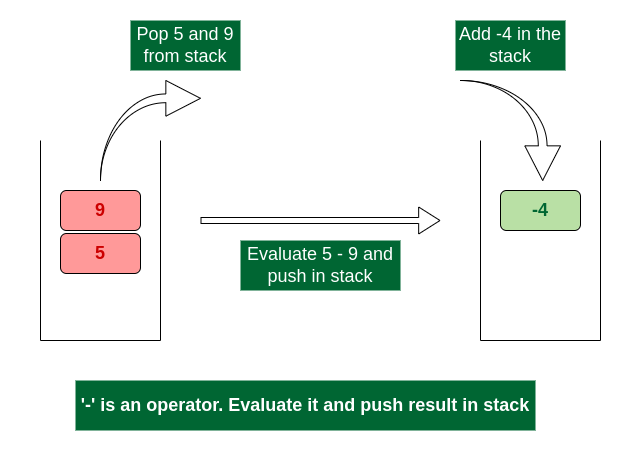Evaluate ‘-‘ operator and push result in stack

• There are no more elements to scan, we return the top element from the stack (which is the only element left in a stack).

So the result becomes -4.

Follow the steps mentioned below to evaluate postfix expression using stack:

• Create a stack to store operands (or values).
• Scan the given expression from left to right and do the following for every scanned element.
• If the element is a number, push it into the stack.
• If the element is an operator, pop operands for the operator from the stack. Evaluate the operator and push the result back to the stack.
• When the expression is ended, the number in the stack is the final answer.

Below is the implementation of the above approach:

## C

 `// C program to evaluate value of a postfix expression` `#include ` `#include ` `#include ` `#include `   `// Stack type` `struct` `Stack {` `    ``int` `top;` `    ``unsigned capacity;` `    ``int``* array;` `};`   `// Stack Operations` `struct` `Stack* createStack(unsigned capacity)` `{` `    ``struct` `Stack* stack` `        ``= (``struct` `Stack*)``malloc``(``sizeof``(``struct` `Stack));`   `    ``if` `(!stack)` `        ``return` `NULL;`   `    ``stack->top = -1;` `    ``stack->capacity = capacity;` `    ``stack->array` `        ``= (``int``*)``malloc``(stack->capacity * ``sizeof``(``int``));`   `    ``if` `(!stack->array)` `        ``return` `NULL;`   `    ``return` `stack;` `}`   `int` `isEmpty(``struct` `Stack* stack)` `{` `    ``return` `stack->top == -1;` `}`   `char` `peek(``struct` `Stack* stack)` `{` `    ``return` `stack->array[stack->top];` `}`   `char` `pop(``struct` `Stack* stack)` `{` `    ``if` `(!isEmpty(stack))` `        ``return` `stack->array[stack->top--];` `    ``return` `'\$'``;` `}`   `void` `push(``struct` `Stack* stack, ``char` `op)` `{` `    ``stack->array[++stack->top] = op;` `}`   `// The main function that returns value ` `// of a given postfix expression` `int` `evaluatePostfix(``char``* ``exp``)` `{` `    ``// Create a stack of capacity equal to expression size` `    ``struct` `Stack* stack = createStack(``strlen``(``exp``));` `    ``int` `i;`   `    ``// See if stack was created successfully` `    ``if` `(!stack)` `        ``return` `-1;`   `    ``// Scan all characters one by one` `    ``for` `(i = 0; ``exp``[i]; ++i) {` `        `  `        ``// If the scanned character is an operand ` `        ``// (number here), push it to the stack.` `        ``if` `(``isdigit``(``exp``[i]))` `            ``push(stack, ``exp``[i] - ``'0'``);`   `        ``// If the scanned character is an operator, ` `        ``// pop two elements from stack apply the operator` `        ``else` `{` `            ``int` `val1 = pop(stack);` `            ``int` `val2 = pop(stack);` `            ``switch` `(``exp``[i]) {` `            ``case` `'+'``:` `                ``push(stack, val2 + val1);` `                ``break``;` `            ``case` `'-'``:` `                ``push(stack, val2 - val1);` `                ``break``;` `            ``case` `'*'``:` `                ``push(stack, val2 * val1);` `                ``break``;` `            ``case` `'/'``:` `                ``push(stack, val2 / val1);` `                ``break``;` `            ``}` `        ``}` `    ``}` `    ``return` `pop(stack);` `}`   `// Driver code` `int` `main()` `{` `    ``char` `exp``[] = ``"231*+9-"``;` `  `  `    ``// Function call` `    ``printf``(``"postfix evaluation: %d"``, evaluatePostfix(``exp``));` `    ``return` `0;` `}`

## C++

 `// C++ program to evaluate value of a postfix expression` `#include ` `using` `namespace` `std;`   `// The main function that returns value ` `// of a given postfix expression` `int` `evaluatePostfix(string ``exp``)` `{` `    ``// Create a stack of capacity equal to expression size` `    ``stack<``int``> st;`   `    ``// Scan all characters one by one` `    ``for` `(``int` `i = 0; i < ``exp``.size(); ++i) {` `        `  `        ``// If the scanned character is an operand ` `        ``// (number here), push it to the stack.` `        ``if` `(``isdigit``(``exp``[i]))` `            ``st.push(``exp``[i] - ``'0'``);`   `        ``// If the scanned character is an operator, ` `        ``// pop two elements from stack apply the operator` `        ``else` `{` `            ``int` `val1 = st.top();` `            ``st.pop();` `            ``int` `val2 = st.top();` `            ``st.pop();` `            ``switch` `(``exp``[i]) {` `            ``case` `'+'``:` `                ``st.push(val2 + val1);` `                ``break``;` `            ``case` `'-'``:` `                ``st.push(val2 - val1);` `                ``break``;` `            ``case` `'*'``:` `                ``st.push(val2 * val1);` `                ``break``;` `            ``case` `'/'``:` `                ``st.push(val2 / val1);` `                ``break``;` `            ``}` `        ``}` `    ``}` `    ``return` `st.top();` `}`   `// Driver code` `int` `main()` `{` `    ``string ``exp` `= ``"231*+9-"``;` `  `  `    ``// Function call` `    ``cout << ``"postfix evaluation: "` `<< evaluatePostfix(``exp``);` `    ``return` `0;` `}`

## Java

 `// Java program to evaluate value of a postfix expression`   `import` `java.util.Stack;`   `public` `class` `Test {` `    `  `    ``// Method to evaluate value of a postfix expression` `    ``static` `int` `evaluatePostfix(String exp)` `    ``{` `        ``// Create a stack` `        ``Stack stack = ``new` `Stack<>();`   `        ``// Scan all characters one by one` `        ``for` `(``int` `i = ``0``; i < exp.length(); i++) {` `            ``char` `c = exp.charAt(i);`   `            ``// If the scanned character is an operand` `            ``// (number here), push it to the stack.` `            ``if` `(Character.isDigit(c))` `                ``stack.push(c - ``'0'``);`   `            ``//  If the scanned character is an operator, pop` `            ``//  two elements from stack apply the operator` `            ``else` `{` `                ``int` `val1 = stack.pop();` `                ``int` `val2 = stack.pop();`   `                ``switch` `(c) {` `                ``case` `'+'``:` `                    ``stack.push(val2 + val1);` `                    ``break``;` `                ``case` `'-'``:` `                    ``stack.push(val2 - val1);` `                    ``break``;` `                ``case` `'/'``:` `                    ``stack.push(val2 / val1);` `                    ``break``;` `                ``case` `'*'``:` `                    ``stack.push(val2 * val1);` `                    ``break``;` `                ``}` `            ``}` `        ``}` `        ``return` `stack.pop();` `    ``}`   `    ``// Driver code` `    ``public` `static` `void` `main(String[] args)` `    ``{` `        ``String exp = ``"231*+9-"``;` `      `  `        ``// Function call` `        ``System.out.println(``"postfix evaluation: "` `                           ``+ evaluatePostfix(exp));` `    ``}` `}` `// Contributed by Sumit Ghosh`

## Python3

 `# Python program to evaluate value of a postfix expression`     `# Class to convert the expression` `class` `Evaluate:`   `    ``# Constructor to initialize the class variables` `    ``def` `__init__(``self``, capacity):` `        ``self``.top ``=` `-``1` `        ``self``.capacity ``=` `capacity` `        `  `        ``# This array is used a stack` `        ``self``.array ``=` `[]`   `    ``# Check if the stack is empty` `    ``def` `isEmpty(``self``):` `        ``return` `True` `if` `self``.top ``=``=` `-``1` `else` `False`   `    ``# Return the value of the top of the stack` `    ``def` `peek(``self``):` `        ``return` `self``.array[``-``1``]`   `    ``# Pop the element from the stack` `    ``def` `pop(``self``):` `        ``if` `not` `self``.isEmpty():` `            ``self``.top ``-``=` `1` `            ``return` `self``.array.pop()` `        ``else``:` `            ``return` `"\$"`   `    ``# Push the element to the stack` `    ``def` `push(``self``, op):` `        ``self``.top ``+``=` `1` `        ``self``.array.append(op)`   `    ``# The main function that converts given infix expression` `    ``# to postfix expression` `    ``def` `evaluatePostfix(``self``, exp):`   `        ``# Iterate over the expression for conversion` `        ``for` `i ``in` `exp:`   `            ``# If the scanned character is an operand` `            ``# (number here) push it to the stack` `            ``if` `i.isdigit():` `                ``self``.push(i)`   `            ``# If the scanned character is an operator,` `            ``# pop two elements from stack and apply it.` `            ``else``:` `                ``val1 ``=` `self``.pop()` `                ``val2 ``=` `self``.pop()` `                ``self``.push(``str``(``eval``(val2 ``+` `i ``+` `val1)))`   `        ``return` `int``(``self``.pop())`       `# Driver code` `if` `__name__ ``=``=` `'__main__'``:` `    ``exp ``=` `"231*+9-"` `    ``obj ``=` `Evaluate(``len``(exp))` `    `  `    ``# Function call` `    ``print``(``"postfix evaluation: %d"` `%` `(obj.evaluatePostfix(exp)))`   `    `  `# This code is contributed by Nikhil Kumar Singh(nickzuck_007)`

## C#

 `// C# program to evaluate value of a postfix expression` `using` `System;` `using` `System.Collections;`   `namespace` `GFG {` `class` `Geek {` `  `  `    ``// Main() method` `    ``static` `void` `Main()` `    ``{` `        ``Geek e = ``new` `Geek();` `        ``e.v = (``"231*+9-"``);` `        ``e.expression();` `        ``Console.WriteLine(``"postfix evaluation: "` `                          ``+ e.answer);` `        ``Console.Read();` `    ``}`   `    ``//'v' is variable to store the string value` `    ``public` `string` `v;`   `    ``public` `string` `answer;` `    ``Stack i = ``new` `Stack();`   `    ``// evaluation method` `    ``public` `void` `expression()` `    ``{` `        ``int` `a, b, ans;` `        ``for` `(``int` `j = 0; j < v.Length; j++)` `        ``{` `            ``String c = v.Substring(j, 1);` `            ``if` `(c.Equals(``"*"``)) {` `                ``String sa = (String)i.Pop();` `                ``String sb = (String)i.Pop();` `                ``a = Convert.ToInt32(sb);` `                ``b = Convert.ToInt32(sa);` `                ``ans = a * b;` `                ``i.Push(ans.ToString());` `            ``}` `            ``else` `if` `(c.Equals(``"/"``)) {` `                ``String sa = (String)i.Pop();` `                ``String sb = (String)i.Pop();` `                ``a = Convert.ToInt32(sb);` `                ``b = Convert.ToInt32(sa);` `                ``ans = a / b;` `                ``i.Push(ans.ToString());` `            ``}` `            ``else` `if` `(c.Equals(``"+"``)) {` `                ``String sa = (String)i.Pop();` `                ``String sb = (String)i.Pop();` `                ``a = Convert.ToInt32(sb);` `                ``b = Convert.ToInt32(sa);` `                ``ans = a + b;` `                ``i.Push(ans.ToString());` `            ``}` `            ``else` `if` `(c.Equals(``"-"``)) {` `                ``String sa = (String)i.Pop();` `                ``String sb = (String)i.Pop();` `                ``a = Convert.ToInt32(sb);` `                ``b = Convert.ToInt32(sa);` `                ``ans = a - b;` `                ``i.Push(ans.ToString());` `            ``}` `            ``else` `{` `                ``i.Push(v.Substring(j, 1));` `            ``}` `        ``}` `        ``answer = (String)i.Pop();` `    ``}` `}` `}`

## Javascript

 ``

Output

`postfix evaluation: -4`

Time Complexity: O(N)
Auxiliary Space: O(N)

### There are the following limitations of the above implementation.

• It supports only 4 binary operators ‘+’, ‘*’, ‘-‘ and ‘/’. It can be extended for more operators by adding more switch cases.
• The allowed operands are only single-digit operands.

## Postfix evaluation for multi-digit numbers:

The above program can be extended for multiple digits by adding a separator-like space between all elements (operators and operands) of the given expression.

Below given is the extended program which allows operands to have multiple digits.

## C

 `// C program to evaluate value of a postfix` `// expression having multiple digit operands` `#include ` `#include ` `#include ` `#include `   `// Stack type` `struct` `Stack {` `    ``int` `top;` `    ``unsigned capacity;` `    ``int``* array;` `};`   `// Stack Operations` `struct` `Stack* createStack(unsigned capacity)` `{` `    ``struct` `Stack* stack` `        ``= (``struct` `Stack*)``malloc``(``sizeof``(``struct` `Stack));`   `    ``if` `(!stack)` `        ``return` `NULL;`   `    ``stack->top = -1;` `    ``stack->capacity = capacity;` `    ``stack->array` `        ``= (``int``*)``malloc``(stack->capacity * ``sizeof``(``int``));`   `    ``if` `(!stack->array)` `        ``return` `NULL;`   `    ``return` `stack;` `}`   `int` `isEmpty(``struct` `Stack* stack)` `{` `    ``return` `stack->top == -1;` `}`   `int` `peek(``struct` `Stack* stack)` `{` `    ``return` `stack->array[stack->top];` `}`   `int` `pop(``struct` `Stack* stack)` `{` `    ``if` `(!isEmpty(stack))` `        ``return` `stack->array[stack->top--];` `    ``return` `'\$'``;` `}`   `void` `push(``struct` `Stack* stack, ``int` `op)` `{` `    ``stack->array[++stack->top] = op;` `}`   `// The main function that returns value` `// of a given postfix expression` `int` `evaluatePostfix(``char``* ``exp``)` `{` `    ``// Create a stack of capacity equal to expression size` `    ``struct` `Stack* stack = createStack(``strlen``(``exp``));` `    ``int` `i;`   `    ``// See if stack was created successfully` `    ``if` `(!stack)` `        ``return` `-1;`   `    ``// Scan all characters one by one` `    ``for` `(i = 0; ``exp``[i]; ++i) {` `        ``// if the character is blank space then continue` `        ``if` `(``exp``[i] == ``' '``)` `            ``continue``;`   `        ``// If the scanned character is an` `        ``// operand (number here),extract the full number` `        ``// Push it to the stack.` `        ``else` `if` `(``isdigit``(``exp``[i])) {` `            ``int` `num = 0;`   `            ``// extract full number` `            ``while` `(``isdigit``(``exp``[i])) {` `                ``num = num * 10 + (``int``)(``exp``[i] - ``'0'``);` `                ``i++;` `            ``}` `            ``i--;`   `            ``// push the element in the stack` `            ``push(stack, num);` `        ``}`   `        ``// If the scanned character is an operator, pop two` `        ``// elements from stack apply the operator` `        ``else` `{` `            ``int` `val1 = pop(stack);` `            ``int` `val2 = pop(stack);`   `            ``switch` `(``exp``[i]) {` `            ``case` `'+'``:` `                ``push(stack, val2 + val1);` `                ``break``;` `            ``case` `'-'``:` `                ``push(stack, val2 - val1);` `                ``break``;` `            ``case` `'*'``:` `                ``push(stack, val2 * val1);` `                ``break``;` `            ``case` `'/'``:` `                ``push(stack, val2 / val1);` `                ``break``;` `            ``}` `        ``}` `    ``}` `    ``return` `pop(stack);` `}`   `// Driver program to test above functions` `int` `main()` `{` `    ``char` `exp``[] = ``"100 200 + 2 / 5 * 7 +"``;` `  `  `    ``// Function call` `    ``printf``(``"%d"``, evaluatePostfix(``exp``));` `    ``return` `0;` `}`   `// This code is contributed by Arnab Kundu`

## C++14

 `// C++ program to evaluate value of a postfix` `// expression having multiple digit operands` `#include ` `using` `namespace` `std;`   `// The main function that returns value` `// of a given postfix expression` `int` `evaluatePostfix(``char``* ``exp``)` `{` `    ``// Create a stack of capacity equal to expression size` `    ``stack<``int``> st;` `    ``int` `i;`   `    ``// Scan all characters one by one` `    ``for` `(i = 0; ``exp``[i]; ++i) {` `        `  `        ``// If the character is blank space then continue` `        ``if` `(``exp``[i] == ``' '``)` `            ``continue``;`   `        ``// If the scanned character is an` `        ``// operand (number here),extract the full number` `        ``// Push it to the stack.` `        ``else` `if` `(``isdigit``(``exp``[i])) {` `            ``int` `num = 0;`   `            ``// Extract full number` `            ``while` `(``isdigit``(``exp``[i])) {` `                ``num = num * 10 + (``int``)(``exp``[i] - ``'0'``);` `                ``i++;` `            ``}` `            ``i--;`   `            ``// Push the element in the stack` `            ``st.push(num);` `        ``}`   `        ``// If the scanned character is an operator, pop two` `        ``// elements from stack apply the operator` `        ``else` `{` `            ``int` `val1 = st.top();` `            ``st.pop();` `            ``int` `val2 = st.top();` `            ``st.pop();`   `            ``switch` `(``exp``[i]) {` `            ``case` `'+'``:` `                ``st.push(val2 + val1);` `                ``break``;` `            ``case` `'-'``:` `                ``st.push(val2 - val1);` `                ``break``;` `            ``case` `'*'``:` `                ``st.push(val2 * val1);` `                ``break``;` `            ``case` `'/'``:` `                ``st.push(val2 / val1);` `                ``break``;` `            ``}` `        ``}` `    ``}` `    ``return` `st.top();` `}`   `// Driver code` `int` `main()` `{` `    ``char` `exp``[] = ``"100 200 + 2 / 5 * 7 +"``;` `  `  `    ``// Function call` `    ``cout << evaluatePostfix(``exp``);` `    ``return` `0;` `}`   `// This code is contributed by rathbhupendra`

## Java

 `// Java program to evaluate value of a postfix` `// expression having multiple digit operands` `import` `java.util.Stack;`   `class` `Test1 {` `    `  `    ``// Method to evaluate value of a postfix expression` `    ``static` `int` `evaluatePostfix(String exp)` `    ``{` `        ``// Create a stack` `        ``Stack stack = ``new` `Stack<>();`   `        ``// Scan all characters one by one` `        ``for` `(``int` `i = ``0``; i < exp.length(); i++) {` `            ``char` `c = exp.charAt(i);`   `            ``if` `(c == ``' '``)` `                ``continue``;`   `            ``// If the scanned character is an operand` `            ``// (number here),extract the number` `            ``// Push it to the stack.` `            ``else` `if` `(Character.isDigit(c)) {` `                ``int` `n = ``0``;`   `                ``// Extract the characters and store it in num` `                ``while` `(Character.isDigit(c)) {` `                    ``n = n * ``10` `+ (``int``)(c - ``'0'``);` `                    ``i++;` `                    ``c = exp.charAt(i);` `                ``}` `                ``i--;`   `                ``// Push the number in stack` `                ``stack.push(n);` `            ``}`   `            ``// If the scanned character is an operator, pop` `            ``// two elements from stack apply the operator` `            ``else` `{` `                ``int` `val1 = stack.pop();` `                ``int` `val2 = stack.pop();`   `                ``switch` `(c) {` `                ``case` `'+'``:` `                    ``stack.push(val2 + val1);` `                    ``break``;` `                ``case` `'-'``:` `                    ``stack.push(val2 - val1);` `                    ``break``;` `                ``case` `'/'``:` `                    ``stack.push(val2 / val1);` `                    ``break``;` `                ``case` `'*'``:` `                    ``stack.push(val2 * val1);` `                    ``break``;` `                ``}` `            ``}` `        ``}` `        ``return` `stack.pop();` `    ``}`   `    ``// Driver program to test above functions` `    ``public` `static` `void` `main(String[] args)` `    ``{` `        ``String exp = ``"100 200 + 2 / 5 * 7 +"``;` `      `  `        ``// Function call` `        ``System.out.println(evaluatePostfix(exp));` `    ``}` `}`   `// This code is contributed by Arnab Kundu`

## Python3

 `# Python program to evaluate value of a postfix` `# expression with integers containing multiple digits`     `class` `evalpostfix:` `    ``def` `__init__(``self``):` `        ``self``.stack ``=` `[]` `        ``self``.top ``=` `-``1`   `    ``def` `pop(``self``):` `        ``if` `self``.top ``=``=` `-``1``:` `            ``return` `        ``else``:` `            ``self``.top ``-``=` `1` `            ``return` `self``.stack.pop()`   `    ``def` `push(``self``, i):` `        ``self``.top ``+``=` `1` `        ``self``.stack.append(i)`   `    ``def` `centralfunc(``self``, ab):` `        ``for` `i ``in` `ab:`   `            ``# If the component of the list is an integer` `            ``try``:` `                ``self``.push(``int``(i))` `            `  `            ``# If the component of the list is not an integer,` `            ``# it must be an operator. Using ValueError, we can` `            ``# evaluate components of the list other than type int` `            ``except` `ValueError:` `                ``val1 ``=` `self``.pop()` `                ``val2 ``=` `self``.pop()` `                ``if` `i ``=``=` `'/'``:` `                    ``self``.push(val2 ``/` `val1)` `                ``else``:` `                    `  `                    ``# Switch statement to perform operation` `                    ``switcher ``=` `{``'+'``: val2 ``+` `val1, ``'-'``: val2 ``-` `                                ``val1, ``'*'``: val2 ``*` `val1, ``'^'``: val2``*``*``val1}` `                    ``self``.push(switcher.get(i))` `        ``return` `int``(``self``.pop())`       `# Driver code` `if` `__name__ ``=``=` `'__main__'``:` `    ``str` `=` `'100 200 + 2 / 5 * 7 +'` `    `  `    ``# Splitting the given string to obtain` `    ``# integers and operators into a list` `    ``strconv ``=` `str``.split(``' '``)` `    ``obj ``=` `evalpostfix()` `    ``print``(obj.centralfunc(strconv))`   `# This code is contributed by Amarnath Reddy`

## C#

 `// C# program to evaluate value of a postfix` `// expression having multiple digit operands` `using` `System;` `using` `System.Collections.Generic;`   `class` `GFG {` `    `  `    ``// Method to evaluate value of` `    ``// a postfix expression` `    ``public` `static` `int` `evaluatePostfix(``string` `exp)` `    ``{` `        ``// Create a stack` `        ``Stack<``int``> stack = ``new` `Stack<``int``>();`   `        ``// Scan all characters one by one` `        ``for` `(``int` `i = 0; i < exp.Length; i++) {` `            ``char` `c = exp[i];`   `            ``if` `(c == ``' '``) {` `                ``continue``;` `            ``}`   `            ``// If the scanned character is an` `            ``// operand (number here),extract` `            ``// the number. Push it to the stack.` `            ``else` `if` `(``char``.IsDigit(c)) {` `                ``int` `n = 0;`   `                ``// Extract the characters and` `                ``// store it in num` `                ``while` `(``char``.IsDigit(c)) {` `                    ``n = n * 10 + (``int``)(c - ``'0'``);` `                    ``i++;` `                    ``c = exp[i];` `                ``}` `                ``i--;`   `                ``// push the number in stack` `                ``stack.Push(n);` `            ``}`   `            ``// If the scanned character is` `            ``// an operator, pop two elements` `            ``// from stack apply the operator` `            ``else` `{` `                ``int` `val1 = stack.Pop();` `                ``int` `val2 = stack.Pop();`   `                ``switch` `(c) {` `                ``case` `'+'``:` `                    ``stack.Push(val2 + val1);` `                    ``break``;`   `                ``case` `'-'``:` `                    ``stack.Push(val2 - val1);` `                    ``break``;`   `                ``case` `'/'``:` `                    ``stack.Push(val2 / val1);` `                    ``break``;`   `                ``case` `'*'``:` `                    ``stack.Push(val2 * val1);` `                    ``break``;` `                ``}` `            ``}` `        ``}` `        ``return` `stack.Pop();` `    ``}`   `    ``// Driver Code` `    ``public` `static` `void` `Main(``string``[] args)` `    ``{` `        ``string` `exp = ``"100 200 + 2 / 5 * 7 +"``;` `      `  `        ``// Function call` `        ``Console.WriteLine(evaluatePostfix(exp));` `    ``}` `}`   `// This code is contributed by Shrikant13`

## Javascript

 ``

Output

`757`

Time Complexity: O(N)
Auxiliary Space: O(N)

My Personal Notes arrow_drop_up